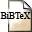# Pairing-Friendly Elliptic Curves of Prime Order

## Authors

P. S. L. M. Barreto, M. Naehrig,

## Abstract

Previously known techniques to construct pairing-friendly curves of
prime or near-prime order are restricted to embedding degree $k \leqslant 6$. More general methods produce curves over $\F_p$ where the
bit length of $p$ is often twice as large as that of the order $r$ of
the subgroup with embedding degree $k$; the best published results
achieve $\rho \equiv \log(p)/\log(r) \sim 5/4$. In this paper we make
the first step towards surpassing these limitations by describing a
method to construct elliptic curves of prime order and embedding degree
$k = 12$. The new curves lead to very efficient implementation:
non-pairing operations need no more than $\F_p^4$ arithmetic, and
pairing values can be compressed to one third of their length in a way
compatible with point reduction techniques. We also discuss the role of
large CM discriminants $D$ to minimize $\rho$; in particular, for
embedding degree $k = 2q$ where $q$ is prime we show that the ability to
handle $\log(D)/\log(r) \sim (q-3)/(q-1)$ enables building curves with
$\rho \sim q/(q-1)$.

## BibTEX Reference Entry@inproceedings{BaNa06,
author = {Paulo Sérgio Licciardi Messeder Barreto and Michael Naehrig},
title = "Pairing-Friendly Elliptic Curves of Prime Order",
pages = "319-331",
booktitle = "Selected Areas in Cryptography: 12th International Workshop, SAC 2005",Download bibtex-file